# Catch up on the latest AI articles# DeepFoids: Simulation Of Fish School Behavior Using Deep Reinforcement Learning

3 main points
✔️ Proposed a method to autonomously acquire fish schooling behavior adapted to various environments by deep reinforcement learning
✔️ Synthetic dataset closely resembling the real one was created using the proposed method and Unity simulations
✔️ Deep learning model successfully trained to count various types of fish in a cove

(Submitted on 01 Nov 2022 (v1), last revised 24 Dec 2022 (this version, v2))The images used in this article are from the paper, the introductory slides, or were created based on them.

## Introduction

The sustainability of food supply and conservation of fishery resources is one of the most important global issues and one that the United Nations must achieve by 2030. With the rapid increase in seafood consumption, aquaculture is one of the sustainable ways to supply seafood to support the world population. Currently, one of the major problems in aquaculture is the management of feed. Low feeding results in malnutrition and slower fish growth while overfeeding can result in residual food contaminating the marine environment. Therefore, it is necessary to automate fish counting using RGB cameras and computer vision to optimize the amount and timing of feeding.

However, there is one problem here. That is that "labeled data sets for learning to measure the number of fish" are difficult to obtain. Therefore, this study proposes a method to autonomously generate fish group behavior using deep reinforcement learning (DRL). This has resulted in multiple distinct collective behavior patterns based on individual density. Then, by creating a high-quality synthetic dataset using environmental simulations with Unity, we successfully trained a deep-learning model that counts the various types of fish in a kayak.

## Fish Simulation

First, the fish behavior model is constructed as a multi-agent reinforcement learning (MARL) problem. Fish agents must swim in a constrained 3D space, similar to a fish farm while considering multiple factors that influence their behavior. In the process of interacting with the environment and other fish, each agent learns to act based on its observations and maximize rewards. In this paper, we use the reinforcement learning method Proximal Policy Optimization (PPO) to optimize the strategy. The figure below outlines the simulation pipeline.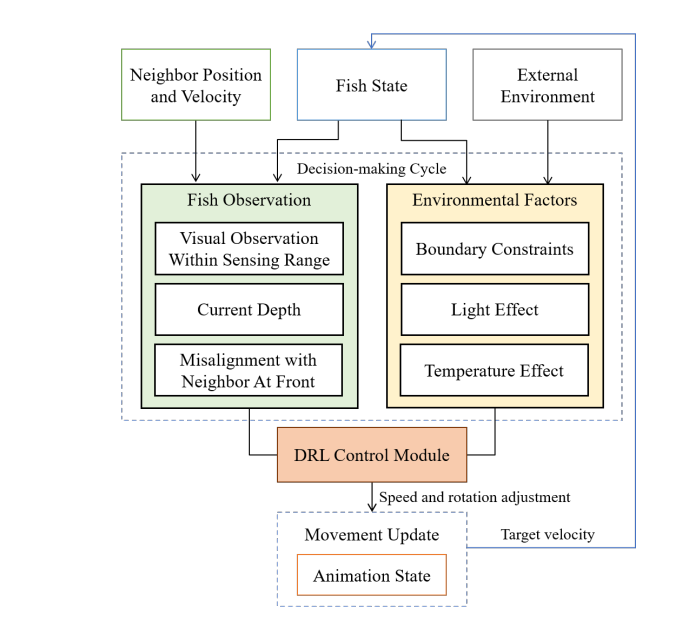### Biological and Environmental Factors

There are important biological and environmental factors that contribute to the swimming patterns of fish. For example, fish stay within a comfortable distance of adjacent fish and align their direction of travel with that of their forward neighbors. Other factors include the fact that fish in the cage is divided into dominant and subordinate groups, with the dominant member aggressively approaching the subordinate member and initiating aggressive behavior. These factors are incorporated into the process of learning the control policy that produces the fish's velocity ∆$v_t^f$ at each time step t (See below for more details.)

Fish also have a preferred range of light levels and water temperatures and will vary their vertical position to stay within their comfortable range. In this paper, they are represented as ∆$v_{light}$$and ∆v_{temp}$$. In addition to the above components, we integrate the decision intervals of the fish into the framework to mimic their delayed response to environmental changes. The simulation decision interval ∆$t_{res}$$is predefined based on previous studies. Given a time interval ∆t_{sim}$$ of simulation steps, the fish updates its observations of the environment at every ⌊∆$t_{res}$/∆$t_{sim}$⌋ step and acts between updates. The cumulative velocity (∆$v_t^a$) to be applied at each simulation step is then derived as follows### States and Actions

Each state $S_t$ is represented by a tuple ($u_t,d_t, I_t$). The $u_t$ is the difference in the forward direction between the agent and the nearest neighbor fish in front of it at the current time step t, $d_t$ is the depth of the agent relative to the water surface, and $I_t$ is the tensor for observations associated with visual information. During its search, the fish agent collects visual observations with spatial grid sensors that mimic the sensing area of a real fish. The visual observation is a cubic tensor whose dimensions are the width of the grid, the height of the grid, and the number of channels. The width and height are defined by the grid resolution, which is set to 34 x 20. There are 6 channels of one-hot encodings of the distance (normalized) from the nearest detected object to the agent within the fish sensing range $d_{sense}$ and the object type (fish, boundary, obstacle, etc.). The $d_{sense}$ is set at 2 body lengths for yellowtail (yellowtail) and 3 body lengths for salmons and tai. All state components are computed in the agent's local coordinate system, with the origin located at the center of the body and the z-axis parallel to the fish's orientation.

The action $a$ is the velocity (∆$v_t$) and the angle of rotation about the x-axis (∆$\theta_t^x$) and y-axis (∆$\theta_t^y$); the angle of rotation about the z-axis is fixed at a small angle $\theta^{zt}$ to avoid unnatural behavior. Also, ∆$v_t$ is fixed at the maximum speed ∆$v_{max}$$allowed in the cage environment. The structure of the network of measures is shown in the figure below.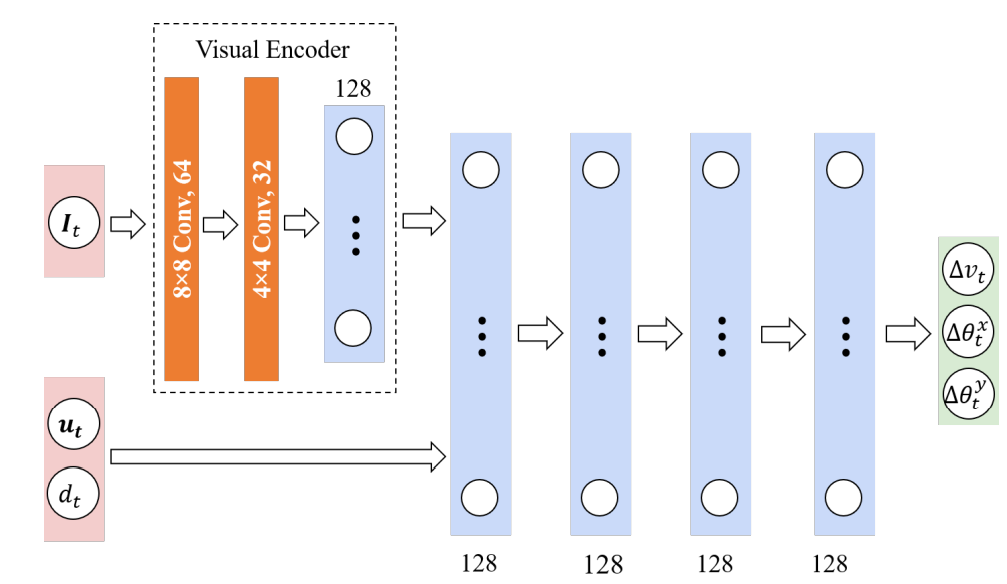### Reward: Reward The reward$r_t$at each time step is defined below to encourage herd behavior while avoiding conflict. Each of these will be explained one at a time.The$r_t^{BC}$represents the penalty due to collisions with spatial boundaries such as cage walls or water surfaces. It is a fixed value of -300 if a boundary collision occurs and 0 otherwise. The$r_t^{NC}$represents the penalty associated with collisions with neighboring fish. It is given using the weight$w^{NC}$and is cumulative with the number of colliding agents$N_{hit}$.The boundary avoidance reward$r_t^{BD}$prompts the agent to distance itself from the detected spatial boundary. Its value depends on the agent's sensing range$d_{sense}$, the number of detected boundaries$N_{bnd}$, the distance to boundary i$d_i$, and the boundary avoidance weight$w^{BD}$.The$R_T^{ND}prompts the fish to approach neighboring fish in its sensing range and align its direction with that of the neighboring fish. The angle ∆\theta_i^{mov}$(in degrees) between the agent and the direction of each of its$N_{nei}$body's neighboring fish, calculated with the weight$w^{ND}$.On the other hand,$r_t^E$penalizes the fish for energy expenditure when it rotates its body or adjusts its velocity. It is calculated from the rotation penalty weight$w^r$, the velocity penalty weight$w^s$, and the angular difference in body rotation ∆$\theta_t$and cumulative velocity difference ∆$v_t^a$at the current time step.The movement rewards$r_t^M$encourages the fish to swim faster than minimum speed and penalizes abrupt depth changes due to aggressive pitch motion (around the local x-axis). In the following equation, the variable$\theta^{rt}$represents the pitch angle threshold,$v^{st}$the velocity threshold,$\theta_t^x$the current pitch angle, and$v_t^a$the current cumulative velocity.Finally,$r_t^C$is a tracking reward that encourages attack or escapes behavior based on the fish's social status. The dominant fish (the attacker) randomly initiates the chase mode with a small probability$p_a$and launches an attack against the nearest dependent fish (the target). This causes the chased fish to initiate escape mode and swim away from the attacker. If the attacker collides with the target, it is rewarded with a fixed, large value. This process is expressed as follows using the attacker's velocity$v_t^a$, the normalized vector d from the attacker to target, the tracking reward weight$w_{agg}$for the attacker, and the escape penalty weight$w_{tar}$for the target.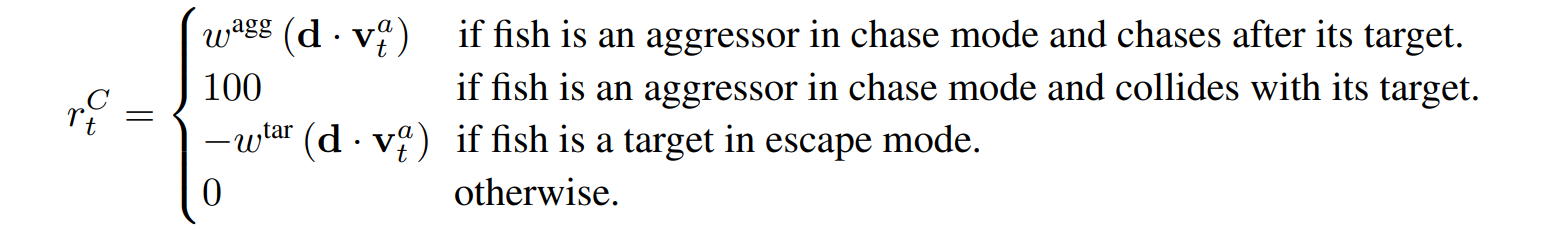## Synthetic Dataset and Fish Counting The simulation is developed in the Unity Engine with the ML-Agents toolkit and utilizes Crest Ocean System HDRP assets to create the effects of the sun and ocean waves. Biological details of the fish, such as species, number, size, and speed, as well as parameters such as temperature and light preference, latitude, longitude, date, and sunlight color and intensity, are present. Existing studies required manual adjustment of parameters when applying simulations to new species or new environments. This study, however, utilizes DRL to train fish to adapt to changing environmental conditions. The figure below is an example of a synthetic dataset.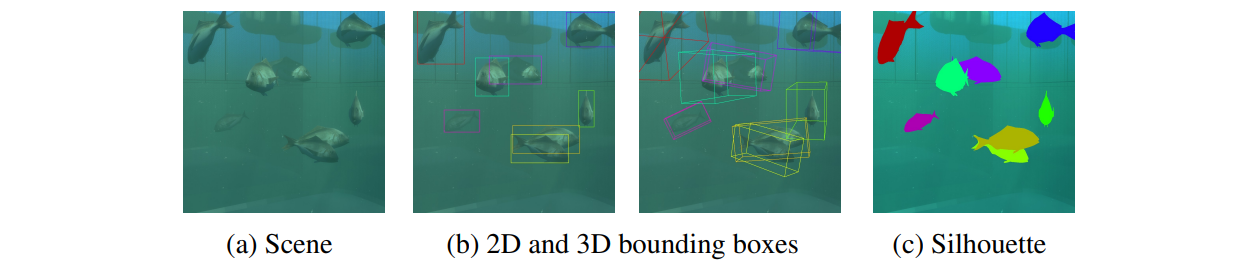The fish counting system consists of three modules: (1) an image preprocessing module that converts the input video into an image sequence and performs noise reduction, (2) a fish detection module that uses a synthetic data set to train a network based on YOLOv4, and (3) a fish counting module. ## Experiments and Results ### Training: Training Three species were used for the study: lingcod, yellowtail, and tai. We first pre-trained each species and then conducted transfer learning in environments with different fish species, fish size, number of fish, cage size, and cage geometry. We found that using this two-stage learning scheme resulted in faster convergence and better overall performance than learning from scratch for each environment. We also allowed the agent to terminate an episode early if it collided with a cage wall or water surface. In that case, the agent resumes a new episode from a random position in the cage with a random effective rotation and initial velocity$v_0$. ### Fish Behavior A qualitative comparison of the images obtained from the simulation and those taken underwater is shown in the figure below. This image shows that the three species of fish swim differently. The simulation successfully reproduced these behavioral differences as well as environmental changes such as lighting, watercolor, and turbidity.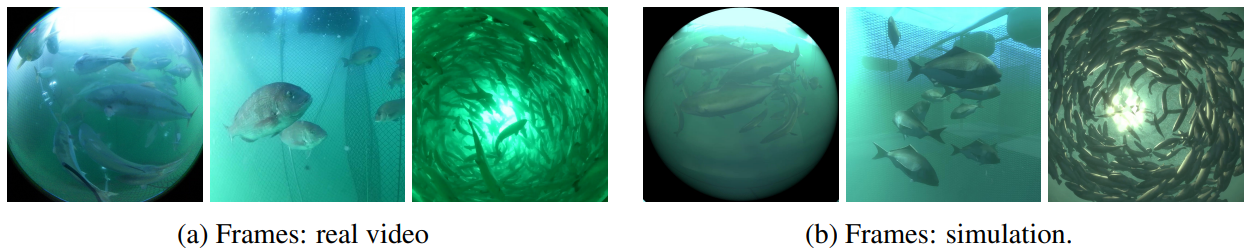Simulation results for five different environments set up for different fish species, fish size, number of fish, cage size, and shape are shown in the figure below. The default body lengths of the lingcod, yellowtail, and snapper were set to 0.49 m, 0.52 m, and 0.34 m, respectively, according to the field data collected. The way fish swim depends on the composition of the scene due to a combination of various factors as just described. For example, the 10 tai in Figure a (left 3 panels) learn to swim slowly around the center of the cage due to the small size of the cage, while the same 10 fish in Figure a (right 3 panels) learn to swim faster away from the center due to the much larger cage size. On the other hand, the tai in Figu reb, because of their larger volume, form a more compact school and slowly circle the entire small cage. We also simulated two fictitious scenes in which fish of different sizes (Figure c) and multiple species (Figure d) are mixed. Fish of different sizes and species swim differently in the same cage, influencing the swimming behavior of other groups.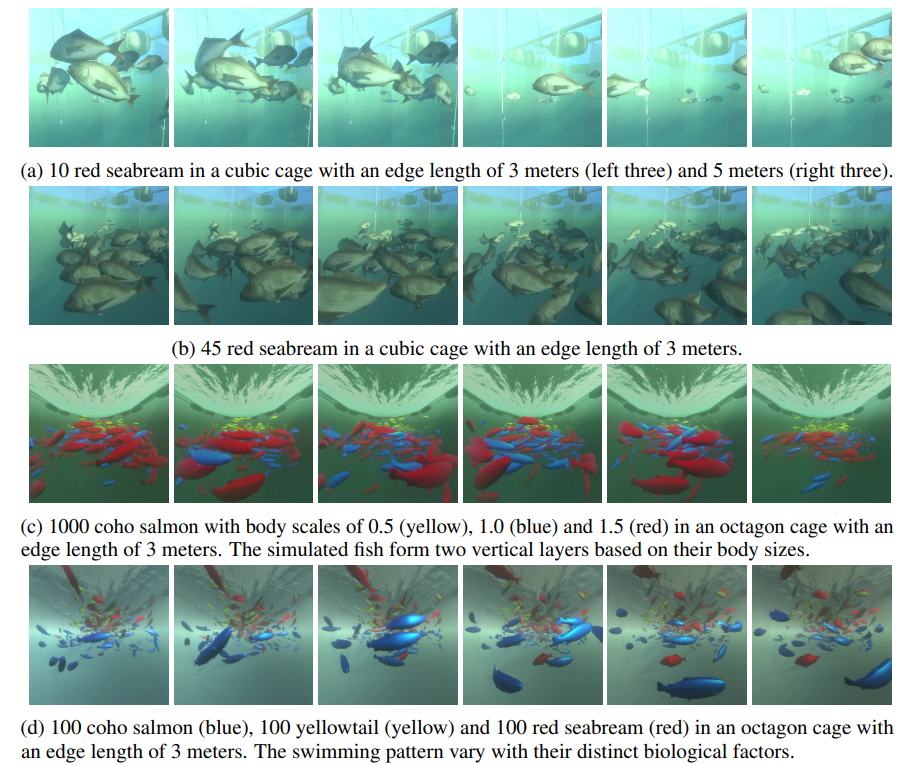### Simulation vs. Reality We compared simulation results with real data, focusing on the relationship between the density of fish in the cage and fish schooling conditions. Two types of cages were used in the simulation: sparse (0.59 fish/$m^3$) and dense (14.7 fish/$m^3\$). In the actual farm, the sparse cage is 6.5m x 6.5m square and 6m deep and holds 272 fish, while the dense cage is an octagonal shape with 6.5m sides and 10m depth and holds about 3,000 fish. The figures below show the trajectories of the fish in both the (a) sparse and (b) dense cases. The left image is the actual image and the right is the result of the simulation.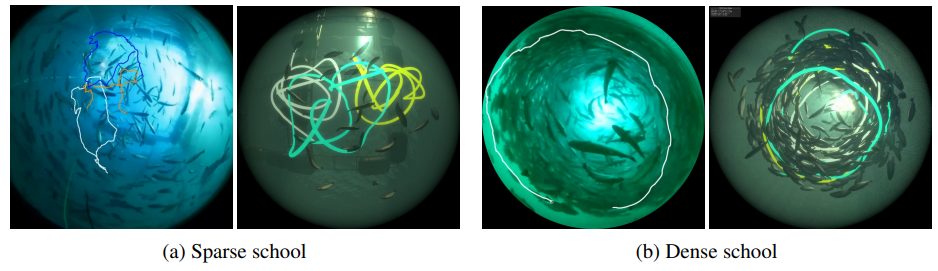### Fish Counting

The YOLOv4 model trained on the synthetic dataset was applied to actual videos. The figure below shows the results using footage of lingcod, yellowtail, and tai. The trained fish counting algorithm was used to estimate the number of fish in each frame of the figure below. The count results for lingcod, yellowtail, and tai were 69:61, 22:32, and 7:7, respectively (left: learned model; right: manual count). This indicates that the accuracy of the counts decreased when fish density was high and occlusion was common.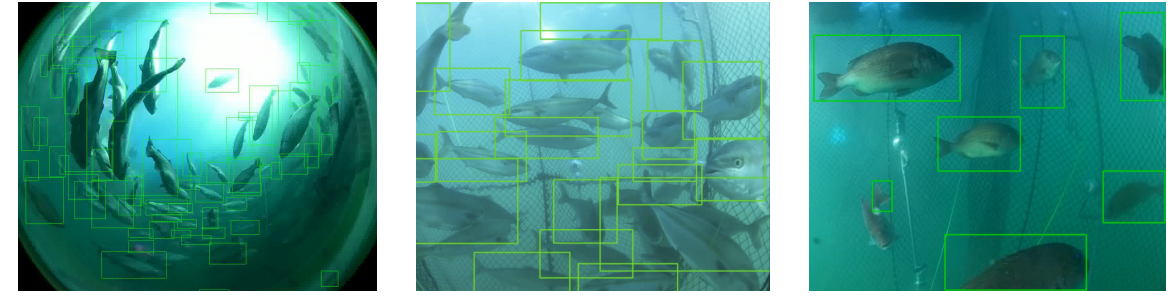## summary

In this presentation, we introduced DeepFoids, a method for autonomously acquiring group behaviors of fish adapted to various environments through deep reinforcement learning. We have achieved the reproduction of swarming behavior using multi-agent DRLs in a given environment. In addition, we incorporated a physics-based simulation that can visually reproduce underwater landscapes in various locations and seasons, allowing us to generate image datasets that closely resemble the real thing.

One challenge is that the weights of the reward function are set manually, and he mentioned that he plans to explore techniques to dynamically adjust the weights, so we look forward to future developments.

If you have any suggestions for improvement of the content of the article,